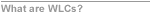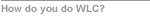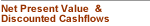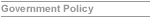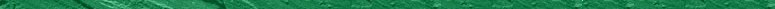﻿ NPV and DCF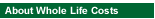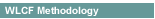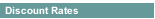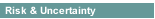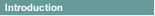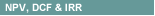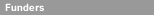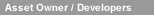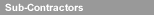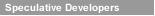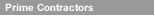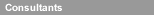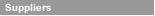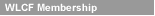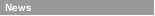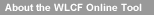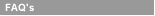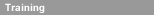Net Present Value & Discounted Cashflows
What are NPV and DCF?
Both NPV (Net Present Value) and DCF (Discounted Cash Flow) are relatively simple accounting concepts, and both use the same principles. NPV and DCF are often used interchangeably.
Why?
Before about 1990 the most common way of selecting between capital projects was a simple payback calculation - how many years of profit would it take to pay back the original investment - which unfortunately did not always lead to the best decisions being taken.
How do NPV and DCF work?
If you were offered 2 similar capital investments of say £100 with payback periods of 2 and 4 years you should accept the first, but if the investments had different life expectations the benefits would be different.
So, investment 1 returns £50 per year but its life is 3 years (or £150), and investment 2 (with only £33 income per year) has a life of 6 years and delivers £200.
The easiest way to explain Net Present Value is in terms of this well understood principle - the return required by an investor.
Let's suppose you have some money to invest, and you have been offered a government bond as an investment (bonds give fixed returns so are sold at a lower amount than the face value). When the bond matures, exactly one year from now, you will be able to exchange it for £100 in cash.
The bank issuing the bond is 100% reliable and risk-free so no risk premium needs to be applied.
So the question is: how much would you be willing to pay now in order to obtain this bond?
Given the choice between receiving £100 today or £100 next year, almost everybody would choose to have the money now.
In other words, money today is more valuable than the same amount of money tomorrow. This principle is called the ‘time value of money’.
The difference in value is not only due to inflation - even if inflation were zero, people would virtually always prefer to receive money now than in a year's time. The main reasons for this are:
 · most people have an expectation that their wealth will be greater in the future, so the relative value to them of a particular sum of money will be correspondingly less in the future
 · money received now can be 'put to work' to earn a return so that, in a year's time it will have accumulated in value
 · the benefit of money received now is certain whereas, because there is no guarantee that you will be alive next year, the benefit of money received next year is uncertain.
Suppose you want to earn 10% annual interest on your investment. You will therefore be willing to pay about £90.91 for the bond.
The present value (PV) is calculated using the formula
PV = The value of the bond x 100/(100 + the required return)
PV = £100 x 100/110
PV = £91.09
We can check that by working the other way:-
£90.91 x 10% = £9.09
and £90.91 + £9.09 = £100 (the cash value of the bond in 12 months)
or put another way £90.91 x 1.10 = £100.
So, at a 10% rate of return, the bond is worth £90.91 today.
Another way of saying the same thing is:-
at a discount rate of 10%, the bond has a present value of £90.91.
This means that, to me today, the value of £100 in 12 months time is £90.91. So, it can be seen how the future value of money is discounted by a rate that is time-related.
Now, consider another investment. This time it is a £1000 bond maturing in 2 years.
But this time you only require an 8% rate of return on your investment. You would therefore be willing to pay the present value today £857.34 for the bond (£857.34 x 1.08 x 1.08 = £1,000).
The present value of a future sum of money therefore depends on three factors:
 · how big the cashflows are
 · how far into the future the sum of money will be paid or received
 · the rate of return that is expected or required?
In present value terms, any future set of cashflows, including inflows and outflows, will have a current value depending on the time-period and discount rate applied.
For any given discount rate, the present value of a future sum of money is the amount you would need to invest today, at an interest rate equal to the discount rate, in order to obtain that sum of money.
The mathematical formula for calculating the present value of an amount of money is:
V = X ÷ (1 + R)N
where V is the present value, at a discount rate of R, of an amount X which is paid or received N years in the future.
How are DCF and NPV used?
In a DCF analysis of a project, each component of the project cash flow is treated as if it were equivalent to a bond - in other words, it is the promise of a particular sum of money at some specific time in the future.
Once you have chosen what discount rate you wish to use, you can calculate the present values of the different parts of the cash flow. If you sum together the present values of all the different components of the project cash-flow, the result is called the Net Present Value.
The problem with projects is that the initial flows are usually costs generating net outflows and that the benefits, or inflows, do not start to accumulate until a later date. The longer that time-period or the lower the inflows the less value they will have today.